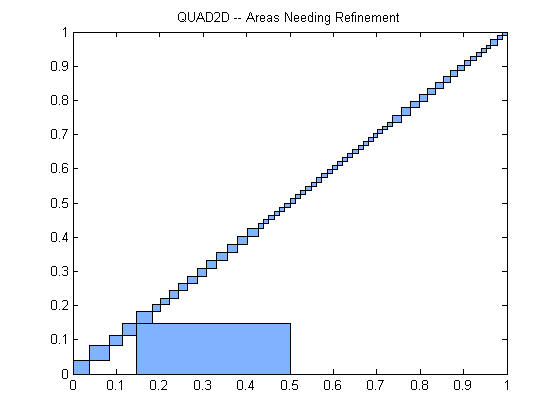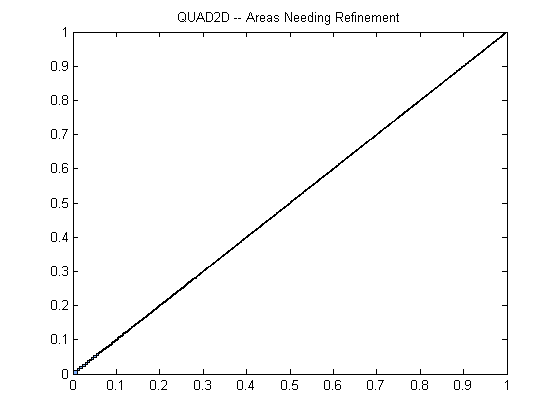Double Integration in MATLAB – Methods and Handling Discontinuities, Singularities, and More

In our recent post, Mike Hosea and I talked about adjusting both the absolute and relative tolerances for getting more accurate results when calculating a double integral. Today we'd like to talk about choosing the method of integration as well as which order to choose for the first dimension (direction) of integration.

Contents

Set the Stage

integral2 has, at present, two different integration methods, 'tiled' and 'iterated', not counting the 'auto' method that chooses between them.

Each integration method employs a type of "divide-and-conquer" approach to double integration but in very different ways. The 'tiled' method is based on quad2d's approach of dividing the region into quadrants and approximating the integral over each quadrant by a 2-D quadrature rule. If the error condition on a rectangle is not met, the rectangle is divided into quadrants and so forth. The 'iterated' method, as the name suggests, performs the integration as iterated one-dimensional integrals, so its "divide-and-conquer" strategy is to tackle one dimension first and then move on to the other.

So, why have two methods? Each has different strengths. Usually the tiled approach is the faster of the two, but it has its weaknesses. As an example of this, consider the way integrating over non-rectangular regions was formerly done in MATLAB. Because dblquad would only integrate over rectangles, one would "mask" the input function to make it zero inside of a rectangular region. For example, to integrate F over a triangle with the vertices (0,0),(1,0), and (1,1), you would write

F = @(x,y)exp(10*x).*tan(y)
F =
@(x,y)exp(10*x).*tan(y)
Q =
1072.6

Q = integral2(F,0,1,0,@(x)x)
Q =
1072.6

This is more than a convenience. Telling the integrator where the boundary is gives it an enormous advantage. Conversely, masking the integrand has devastating effects on performance and accuracy. The underlying quadrature rule gives the best results when the integrand is smooth, and a discontinuity where the integrand drops precipitously to zero will cause the adaptive quadrature algorithm to subdivide and further subdivide in that area until the error estimates across the discontinuity are sufficiently small.

This is especially problematic for quad2d and integral2 with the 'tiled' method. With the 'iterated' method, any curvilinear discontinuity is covered by a single interval in any given integration, but in two dimensions, subdividing each tile into 4 pieces, the integrator ends up covering a curvilinear path with a large number of small rectangles. Usually the limit on the number of function evaluations will be reached before it can polish them all off.

We can visualize this using quad2d's FailurePlot option and use the MaxFunEvals option to control how much work is done before the code gives up. Suppose

F = @(x,y)sin(x./(y.*y + 1)).*(y <=x)
F =
@(x,y)sin(x./(y.*y+1)).*(y<=x)

Then

Warning: Reached the maximum number of function evaluations (60). The result
fails the global error test.
Q =
0.26562There's nothing special about the larger rectangle on the bottom. quad2d preferentially attacks the rectangles with the largest error, so when a larger rectangle just barely fails the error test, it hangs around if the integrator has bigger problems elsewhere. Those bigger problems are signified by smaller rectangles. Now, if we increase the number of allowed function evaluations, we see that quad2d is still struggling with the boundary.

Warning: Reached the maximum number of function evaluations (600). The result
fails the global error test.
Q =
0.26518Since integral2's 'tiled' method is based on quad2d's algorithm, it has the same issue.

Q = integral2(F,0,1,0,1)
Warning: Reached the maximum number of function evaluations (10000). The result
fails the global error test.
Q =
0.26514

Although it takes some time to do it, the 'iterated' method can deal with the problem.

tic
Q = integral2(F,0,1,0,1,'method','iterated')
tq = toc
Q =
0.26513
tq =
5.0793

If you find that the 'iterated' method seems slow, consider loosening the tolerances. integral2 uses the same tolerances for all subintegrations, and it turns out that this can be a very conservative thing to do. With the default tolerances, the previous example took over 4 seconds on the machine we publish the blog from.

tic
Q = integral2(F,0,1,0,1,'method','iterated','AbsTol',1e-6,'RelTol',1e-3)
tq(2) = toc
Q =
0.26514
tq =
5.0793      0.14705

And that took less than a tenth of second on the same machine.

Changing the Order of Integration

Discontinuities may snake through the region of integration, but occasionally they might be linear and parallel to one axis or the other. In that case the order of integration matters when using the 'iterated' method. Since every outer integration performs many inner integrations, it tends to be more useful to make the outer integrations the more efficient ones if possible, and this means letting the inner integrations take care of jump discontinuities. In this example the integrand function alternates between z = 0 and z = y depending on whether floor(x) is even or odd.

f = @(x,y)mod(floor(x),2).*y
tic
integral2(f,0,20,0,1,'method','iterated')
toc
f =
@(x,y)mod(floor(x),2).*y
ans =
5
Elapsed time is 5.625905 seconds.

That worked, but the "inner" integration is in the y-direction, and for a given x that means that the function is either identically zero or a straight line. Either is very easy to integrate. However, this means that the integrand for the outer integral has jump discontinuities from 0 to 0.5 depending on whether floor(x) is even or odd. We'd rather have the inner integrals deal with any (or at least most of) the discontinuities. Changing the order of integration is easy.

tic
integral2(@(y,x)f(x,y),0,1,0,20,'method','iterated')
toc
ans =
5
Elapsed time is 0.264443 seconds.

Singularities

Unlike dblquad, integral2 can integrate singularities on the boundary if they are not too severe. If your problem has a singularity in the interior of the region of integration, you should divide the integral into pieces so that all singularities reside on the boundaries of regions of integration. For example,

f = @(x,y)1./sqrt(abs(x.*y))
f =
@(x,y)1./sqrt(abs(x.*y))

If we integrate this over the square -1 <= x <= 1, -1 <= y <= 1, we have a problem because f is singular along both x and y axes. Obviously f is symmetric with respect to both axes, so we need only integrate over 0 <= x <= 1, 0 <= y <= 1 and multiply by 4, but let's set this aside and try to do it directly.

integral2(f,-1,1,-1,1,'RelTol',1e-12,'AbsTol',1e-14)
Warning: Reached the maximum number of function evaluations (10000). The result
fails the global error test.
ans =
15.869

The 'iterated' method doesn't bail us out this time.

integral2(f,-1,1,-1,1,'RelTol',1e-12,'AbsTol',1e-14,'Method','iterated')
Warning: Reached the limit on the maximum number of intervals in use.
Approximate bound on error is   9.0e-09. The integral may not exist, or it may
be difficult to approximate numerically to the requested accuracy.
Warning: The integration was unsuccessful.
ans =
NaN

However if we split the integration into four pieces, putting the singularity on the boundary, the problem is absolutely routine.

Q = 0;
Q = Q + integral2(f,-1,0,-1,0,'RelTol',1e-12,'AbsTol',1e-14);
Q = Q + integral2(f,-1,0,0,1,'RelTol',1e-12,'AbsTol',1e-14);
Q = Q + integral2(f,0,1,-1,0,'RelTol',1e-12,'AbsTol',1e-14);
Q = Q + integral2(f,0,1,0,1,'RelTol',1e-12,'AbsTol',1e-14)
Q =
16

Can You Take Advantage of these New Integration Routines?

What kinds of functions do you need to integrate? Will the flexibility from integral2 and it's other companions help you? Let us know here.

Published with MATLAB® R2013b

|